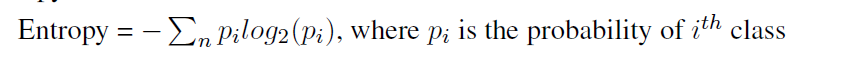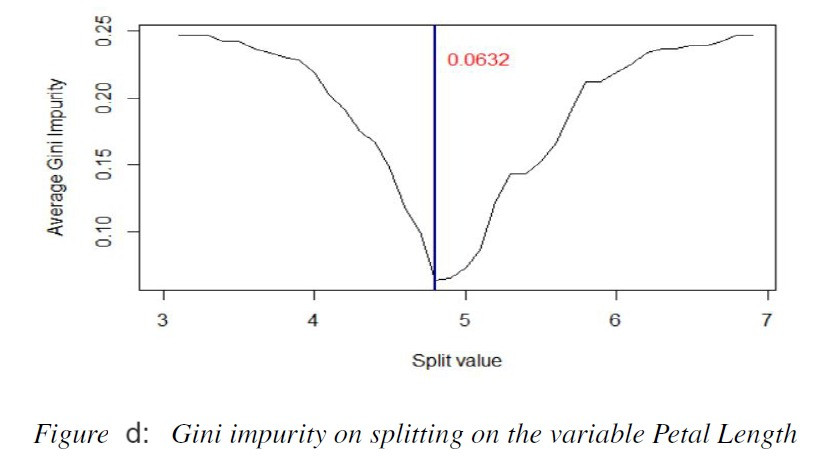Search
•Ekta Aggarwal

# Working of Decision Trees

To understand what is a decision tree let us consider an example:

Suppose a bank wants to decide, whether they want to give loan to a customer they have a few set of rules:

Rule 1 : People who do not have credit history will not be given the loan.

Rule 2: Those people who have the credit history, their income will be checked:

Rule 2 a : If they have credit history and their income is above \$50,000 they will be sanctioned loan.

Rule 2 b : If they have credit history and their income is below \$50,000 their loan will not be approved.

In this scenario we have if-else conditions based on the factors: credit history and income. In other words, if a person has credit history and satisfies a particular income threshold, then their loan will be approved, otherwise rejected.

In real life, these if-else scenarios can be created on large number of variables, thus, we can visually represent them in a decision tree. A decision tree comprises of three main parts, which can

be seen in Figure 2.3:

Root Node: The top node is the root node which is the considered as the best node for

splitting.

Decision Node: These are the nodes where the features are tested and each branch speaks

for the outcome of the test.

Terminal Node / Leaves: These nodes constitute the final decision as the output.

In figure 2.3, the label Petal Width < 1.8 as Yes denote the left-hand branch emanating due to

split of Petal Width, while No represents the right-hand branch corresponding to Petal Width

>= 1.8.Decision Trees divide the input space into a set of rectangles and fit a simple model in each one

of the rectangles (Figure (a)). The tree corresponding to the 2-D feature space partitioned by these rectangles is exhibited by Figure (b). The figure below is due to Hastie, Tibshirani & Friedman (2017).

It checks if X1 <= t1: If yes, then checks is X2 <= t2, If yes then R1, otherwise R2. No further checks are then applied on X1 <= t1

If X1 > t1, then it checks, if X1 <= t3, If yes then R3.

If X1 > t2, and X1 > t3, then it checks if X2 <= t4 then R4, otherwise R5.For classification problems, to find the best feature to split the node and the point of best

split, we use metrics such as entropy or Gini index to assess how much the child nodes are

homogeneous.

### Entropy

Entropy is calculated as:It lies between 0 and 1. If all the pi’s are near 0 or near 1 then entropy will take value 0 (as

log(1) = 0), indicating it is a homogeneous set. For a binary classification problem, if p = 0.5

then entropy will be maximum i.e., 1 which indicates that we have no knowledge of the system.

### Gini Impurity

An alternative to Entropy is Gini Index. It lies between 0 and (1 - 1/n); where n is the number

of categories of dependent variable.

Gini index is calculated as:Gini index of 0 indicates perfect classification, while (1 - 1/n) indicates the worst classification.

The variable having lower Gini index is considered for splitting the node.

### How to decide the best variable and best point to split?

While creating a decision tree, the most obvious question can be : Which is the first variable we should use for splitting? Another question can be: if it is a numerical variable then which is the best value for the split (eg. If income is the best variable then should we consider income <= 40,000 or income <= 50,000 or any other value as our split point). The simple answer is: choose the variable and split point having least Gini Index or Entropy.

To illustrate this, for iris data from UCI Machine Learning Repository, we have created our own Decision Tree. For ease of analysis, we have considered only 2 species: Versicolor and Virginica.

Figures c and d represent the Gini Index at various points for two variables: Petal.Width and

Petal.Length, if they are considered for splitting the root node.

If Petal Width is considered for splitting then, a minimum Gini index of 0.0552 is achieved for Petal Width < 1.7 (Figure c) while using Petal Length would lead to a Gini index of 0.0632 for cut off point 4.8 (Figure d). Thus, for this Petal Width with split point 1.7 is used for splitting the tree.

Further, for cases where Petal Width < 1.7, if we split the decision node by Petal Length, we achieve a minimum Gini index of 0.0428 for splitting point 5 (Figure e).For a regression problem, the best split is considered which minimises the residual sum of### Tuning a Decision Tree:

To find the best Decision Tree there are majorly three parameters to tune:

Maximum depth of the tree: It is extremely essential to decide the appropriate length

(depth) of a Decision Tree. Trees with smaller depth can lead to underfitting while extremely

deep trees can overfit the data.

Minimum number of samples to split: While splitting a node for the Decision Tree, it

must have minimum number of samples to split. A small node size can overfit the data,

while a large node size can underfit the data.

Minimum number of samples per leaf: It is possible that while splitting a node with p

observations, one branch might end up having p - 1 instances and 1 on the other branch.

Thus, we need to tune the minimum number of samples which should be there in the child

node after the parent node is split.

Tags:

See All